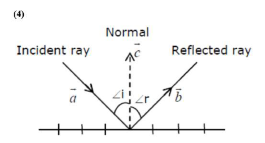# The incident ray, reflected ray and the outward drawn normal are denoted byQuestion:

The incident ray, reflected ray and the outward drawn normal are denoted by the unit vectors $\bar{a}, \vec{b}$ and $\vec{c}$ respectively. Then choose the correct relation for these vectors.

1. (1) $\vec{b}=2 \vec{a}+\vec{c}$

2. (2) $\vec{b}=\vec{a}-\vec{c}$

3. (3) $\vec{b}=\vec{a}+2 \vec{c}$

4. (4) $\vec{b}=\vec{a}-2(\vec{a} \cdot \vec{c}) \vec{c}$

Correct Option: , 4

Solution:We see from the diagram that because of the law of reflection, the component of the unit vector $\vec{a}$ along $\vec{b}$ changes sign on reflection while the component parallel to the mirror remain unchanges, $\vec{a}=\overrightarrow{a_{11}}+\overrightarrow{a_{1}}$

and $\overrightarrow{a_{\perp}}=\vec{c}(\vec{a} \cdot \vec{c})$

we see that the reflected unit vector is $\vec{b}=\overrightarrow{a_{11}}-\overrightarrow{a_{\perp}} \Rightarrow \vec{a}-2(\vec{a} \cdot \vec{c}) \vec{c}$